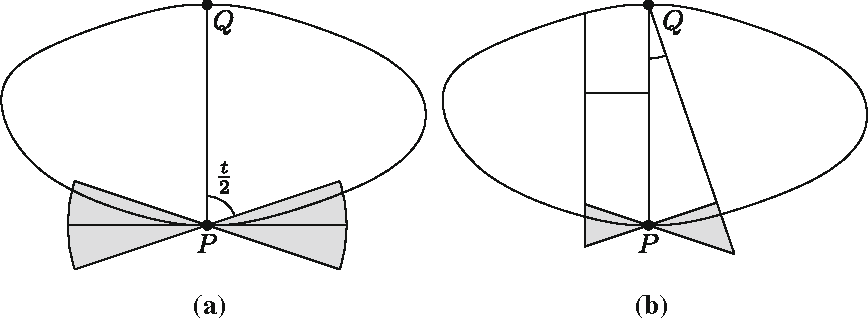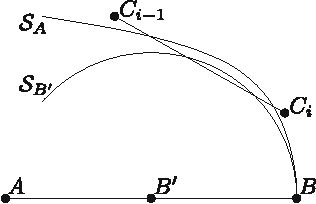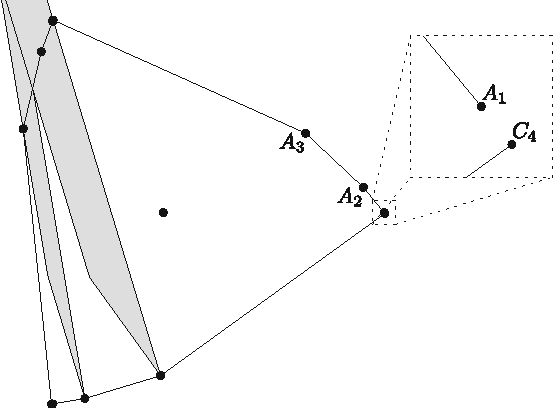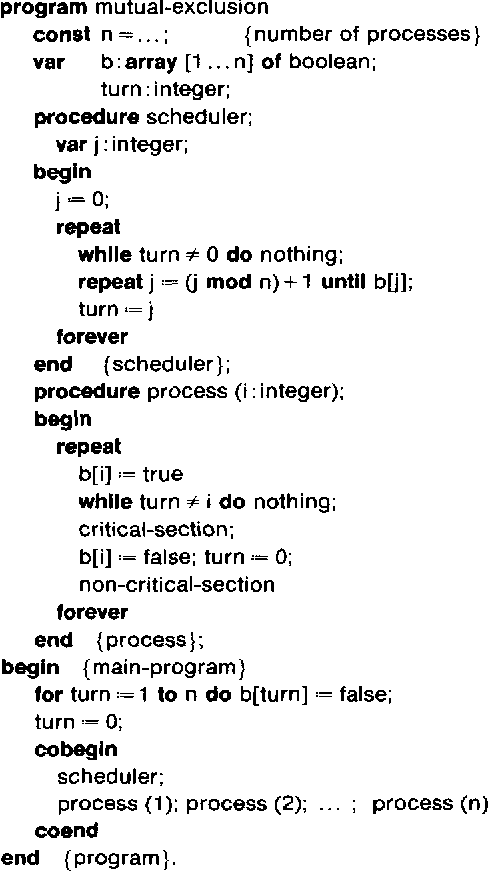Skip to search formSkip to main content
You are currently offline. Some features of the site may not work correctly.

# De Bruijn–Erdős theorem (graph theory)

Known as: De Bruijn-Erdős theorem (graph coloring), De Bruijn–Erdős theorem (graph coloring), De Bruijn
In graph theory, the De Bruijn–Erdős theorem, proved by Nicolaas Govert de Bruijn and Paul Erdős (), states that, for every infinite graph G and… Expand
Wikipedia

## Papers overview

Semantic Scholar uses AI to extract papers important to this topic.
2015
2015
An additive system for the nonnegative integers is a family $$(A_i)_{i\in I}$$ of sets of nonnegative integers with \(0 \in A_i… Expand
2013
2013
• Discret. Comput. Geom.
• 2013
• Corpus ID: 36005038
A well-known theorem of de Bruijn and Erdős states that any set of $$n$$ non-collinear points in the plane determines at least… Expand
2013
2013
• Discret. Comput. Geom.
• 2013
• Corpus ID: 9027525
Given a convex body $$K$$K, consider the smallest number $$N$$N so that there is a point $$P\in \partial K$$P∈∂K such that every… Expand
•••2012
2012
• Des. Codes Cryptogr.
• 2012
• Corpus ID: 15064936
Fix integers n ≥ r ≥ 2. A clique partition of $${{[n] \choose r}}$$ is a collection of proper subsets {A_1, A_2, \ldots, A_t… Expand
2008
2008
• Discret. Appl. Math.
• 2008
• Corpus ID: 1781025
De Bruijn and Erdos proved that every noncollinear set of n points in the plane determines at least n distinct lines. We suggest… Expand
2004
2004
• 2004
• Corpus ID: 17982020
In his 1964 paper, de Bruijn (Math. Comp. 18 (1964) 537) called a pair ða; bÞ of positive odd integers good, if Z ¼ aS~2bS; where… Expand
2002
2002
In 1961, Erdős-Ginzburg-Ziv proved that for a given natural number n ≥ 1 and a sequence a1, a2, · · · , a2n−1 of integers (not… Expand
1995
1995
• Des. Codes Cryptogr.
• 1995
• Corpus ID: 117880
AbstractA De Bruijn torus is a periodicd-dimensionalk-ary array such that eachn1 × ... ×ndk-ary array appears exactly once with… Expand
1984
1984
• Inf. Process. Lett.
• 1984
• Corpus ID: 11861236
In 1965, Dijkstra  published an ingenious solution of the mutual exclusion problem for multiple concurrent processes. The only… Expand
•1982
1982
De Bruijn and Erdős proved that ifA1, ...,Ak are distinct subsets of a set of cardinalityn, and |Ai ∩Aj|≦1 for 1≦in, then some… Expand